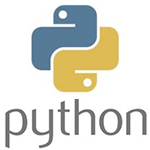# Python教程

Python是一种简单易学，功能强大的编程语言。它包括了高效的高级数据结构和简单而有效的方法，面向对象编程。Python优雅的语法，动态类型，以及它天然的解释能力，使其成为理想的语言，脚本和应用程序快速开发在大多数平台上的许多领域。

Python解释器及其扩展标准库的源码和编译版本可以从Python的Web站点，http://www.python.org/ 所有主要平台可自由查看，并且可以自由发布。该站点上也包含了分配和指针到很多免费的第三方Python模块，程序，工具，以及附加的文档。

Python的解释器很容易扩展新的功能，并在C或C ++（或由C来调用其他语言）实现的数据类型。 Python也很适于作为定制应用的一种扩展语言。

## 1、Python环境安装配置

Python是一种通用的计算机编程语言，跻身排名于世界前八最流行的编程语言。

（在Python2.x中，打印不必使用中括号，对于 Python3.X 是必需的）

```Hello World!
This is a Python program```

## 2、Python 数字

• 自然数（也称为整数或短int）
• 实数（浮点或浮点数）
• 非常大的数字（long）
• 复数（你可能知道，数学课里的复数）

```#!/usr/bin/python

x = 3              # an integer stored (in variable x)
f = 3.1415926      # a floating real point (in variable f)
name = "Python"    # a string
big = 358315791L   # long, a very large number
z = complex(2,3)   # (2+3i)  a complex number. consists of real and imaginary part.

print(x)
print(f)
print(name)
print(big)
print(z)```

```3
3.1415926
Python
358315791
(2+3j)```

signed int -2147483647 2147483647
long - 只受内存限制
float 2.2250738585072014e-308 1.7976931348623157e+308

signed int -9223372036854775807 9223372036854775807
long - 只受内存限制
float 2.2250738585072014e-308 1.7976931348623157e+308

```#!/usr/bin/env python

x = 3
y = 8

sum = x + y

print(sum)```

`11`

```#!/usr/bin/env python

x = int(input("Enter x:"))
y = int(input("Enter y:"))

sum = x + y
print(sum)```

# 3、Python3 字符串

Python已经在内置支持用于存储和操作文本：字符序列被称为子符串。 要定义字符串应将文本放在引号之间，如果使用单引号（'），双引号（"）或三引号（"""），这并不重要。并无规定最少和最大在字符串中可存储字符的数目。一个空字符串没有文字引号。 例如：

```s = 'hello'
s = "hello"
s = """hello"""```

```#!/usr/bin/env python

s = "Hello world" # define the string
print(s)
print(len(s)) # prints the length of the string```

## 字符串的索引

Python索引字符串的字符，每一个索引与一个唯一的字符相关联。例如，在字符串“python”中的字符的索引：Python字符串的索引

```#!/usr/bin/python

s = "Hello Python"
print(s)      # prints whole string
print(s)   # prints "H"
print(s) # prints "e"```

## 字符串切片

`s[ startIndex : pastIndex ]`
startIndex是字符串的开始索引。pastIndex是切片的终点。如果省略了第一个索引，切片将从头开始。如果省略了最后一个索引，切片则到字符串的结尾。 例如：
```#!/usr/bin/python

s = "Hello Python"
print(s[0:2]) # prints "He"
print(s[2:4]) # prints "ll"
print(s[6:])  # prints "Python"
```

## 修改字符串

```#!/usr/bin/python

s = "Hello Python"

print(s + ' ' + s) # print concatenated string.
print(s.replace('Hello','Thanks')) # print a string with a replaced word

# convert string to uppercase
s = s.upper()
print(s)

# convert to lowercase
s = s.lower()
print(s)```

## Python字符串比较，包含和串联

```#!/usr/bin/python

sentence = "The cat is brown"
q = "cat"

if q == sentence:
print('strings equal')

if q in sentence:
print(q + " found in " + sentence)```

## 转义字符

```#!/usr/bin/env python

str1 = "In Python,\nyou can use special characters in strings.\nThese special characters can be..."
print(str1)```

```#!/usr/bin/env python

str1 = "The word \"computer\" will be in quotes."
print(str1)```

# 4、Python 列表

Python有一个数据类型叫列表，被称为 ‘list’.   列表可以包含字符串（文字）和数字。列表使用的是括号定义 [].  要访问数据，使用相同的括号。如字符串，第一元素是。列表用法示例如下：

```#!/usr/bin/python

l = [ "Drake", "Derp", "Derek", "Dominique" ]

print l     # prints all elements
print l  # print first element
print l  # prints second element```

```['Drake', 'Derp', 'Derek', 'Dominique']
Drake
Derp```

## 追加和删除列表项

```#!/usr/bin/python

l = [ "Drake", "Derp", "Derek", "Dominique" ]

print l                # prints all elements
print l                # print all elements
l.remove("Derp")       # remove element.
l.remove("Drake")      # remove element.
print l                # print all elements.```

```['Drake', 'Derp', 'Derek', 'Dominique']
['Drake', 'Derp', 'Derek', 'Dominique', 'Victoria']
['Derek', 'Dominique', 'Victoria']```

## 列表排序

```#!/usr/bin/python

l = [ "Drake", "Derp", "Derek", "Dominique" ]

print l     # prints all elements
l.sort()    # sorts the list in alphabetical order
print l     # prints all elements```

```['Drake', 'Derp', 'Derek', 'Dominique']
['Derek', 'Derp', 'Dominique', 'Drake']```

```#!/usr/bin/python

l = [ "Drake", "Derp", "Derek", "Dominique" ]

print l     # prints all elements
l.sort()    # sorts the list in alphabetical order
l.reverse() # reverse order.
print l     # prints all elements```

```['Drake', 'Derp', 'Derek', 'Dominique']
['Drake', 'Dominique', 'Derp', 'Derek']```

# 5、Python 元组

`tuple = ()`

`tuple = (3,)`

`personInfo = ("Diana", 32, "New York")`

```#!/usr/bin/env python

personInfo = ("Diana", 32, "New York")
print(personInfo)
print(personInfo)```

```Diana
32```

```#!/usr/bin/env python

print(country)```

`Canada`

## Python中追加元素到元组

```#!/usr/bin/env python

x = (3,4,5,6)
x = x + (1,2,3)
print(x)```

`(3, 4, 5, 6, 1, 2, 3)`

## 转换元组

```#!/usr/bin/env python

listNumbers = [6,3,7,4]
x = tuple(listNumbers)
print(x)```

```#!/usr/bin/env python

x = (4,5)
listNumbers = list(x)
print(listNumbers)```

```#!/usr/bin/env python

s = ' '.join(person)
print(s)```

## 排序元组

```#!/usr/bin/env python

person = ('Alison','Victoria','Brenda','Rachel','Trevor')
person = tuple(sorted(person))
print(person)```

# 6、Python3 字典

```#!/usr/bin/python

words = {}
words["Hello"] = "Bonjour"
words["Yes"] = "Oui"
words["No"] = "Non"
words["Bye"] = "Au Revoir"

print words["Hello"]
print words["No"]```

```Bonjour
Non```

```#!/usr/bin/python

dict = {}
dict['Ford'] = "Car"
dict['Python'] = "The Python Programming Language"
dict = "This sentence is stored here."

print dict['Ford']
print dict['Python']
print dict```

```Car
The Python Programming Language
This sentence is stored here.```

```#!/usr/bin/python

words = {}
words["Hello"] = "Bonjour"
words["Yes"] = "Oui"
words["No"] = "Non"
words["Bye"] = "Au Revoir"

print words            # print key-pairs.
del words["Yes"]       # delete a key-pair.
print words            # print key-pairs.
words["Yes"] = "Oui!"  # add new key-pair.
print words            # print key-pairs.```

```{'Yes': 'Oui', 'Bye': 'Au Revoir', 'Hello': 'Bonjour', 'No': 'Non'}
{'Bye': 'Au Revoir', 'Hello': 'Bonjour', 'No': 'Non'}
{'Yes': 'Oui!', 'Bye': 'Au Revoir', 'Hello': 'Bonjour', 'No': 'Non'}```